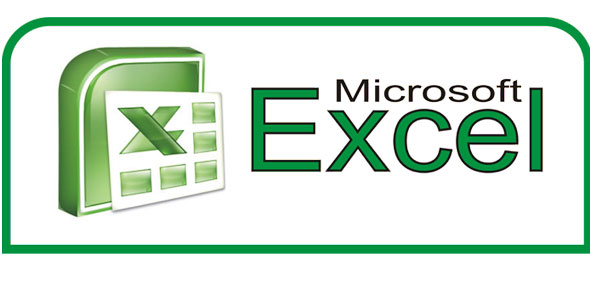# Understanding Microsoft Excel For Grade: 9mj

15 Questions | Attempts: 446
ShareSettingsClass test

• 1.
• A.

A game we play in class

• B.

Fill with lines and we just play with them

• C.

This is a piece of software that make it easy for you to carry out calclations

• D.

A software used for many task that are important to the boot up

• 2.
ALL is a part of Microsoft excel EXCEPT ?
• A.

Active cell

• B.

• C.

Window file

• D.

Formula bar

• 3.
Which is correct about CHARTS in Microsoft excel?
• A.

they are visual representation of the worksheet data

• B.

Maps to the world

• C.

Graphics

• D.

Designs

• 4.
Row heading are rows labeled with numbers, starting with 1
• A.

True

• B.

False

• 5.
A Range is a group of books of calls in a workstation
• A.

True

• B.

False

• 6.
Value contains numbers and can be used in calculations
• A.

True

• B.

False

• 7.
Start a formula with ___________________________ ?
• 8.
One box in a spreadsheet. The place where a row and a column intersect
• A.

Cell

• B.

Row

• C.

Column

• D.

Alignment

• 9.
A computer program with Rows, columns, cells that lets you organize information and do math
• A.

Autosum

• B.

Descending

• C.

Format

• D.

• 10.
The horizontal space (across) that has numbers on the left side
• A.

Column

• B.

Cell

• C.

Allignment

• D.

Row

• 11.
Tell how  you would open Microsoft excel ?
• 12.
AVERAGE  is used to find the size of the smallest class
• A.

True

• B.

False

• 13.
MAX is used to show the size of the largest class
• A.

True

• B.

False

• 14.
MIN is used to show the average class size
• A.

True

• B.

False

## Related TopicsBack to top
×

Wait!
Here's an interesting quiz for you.